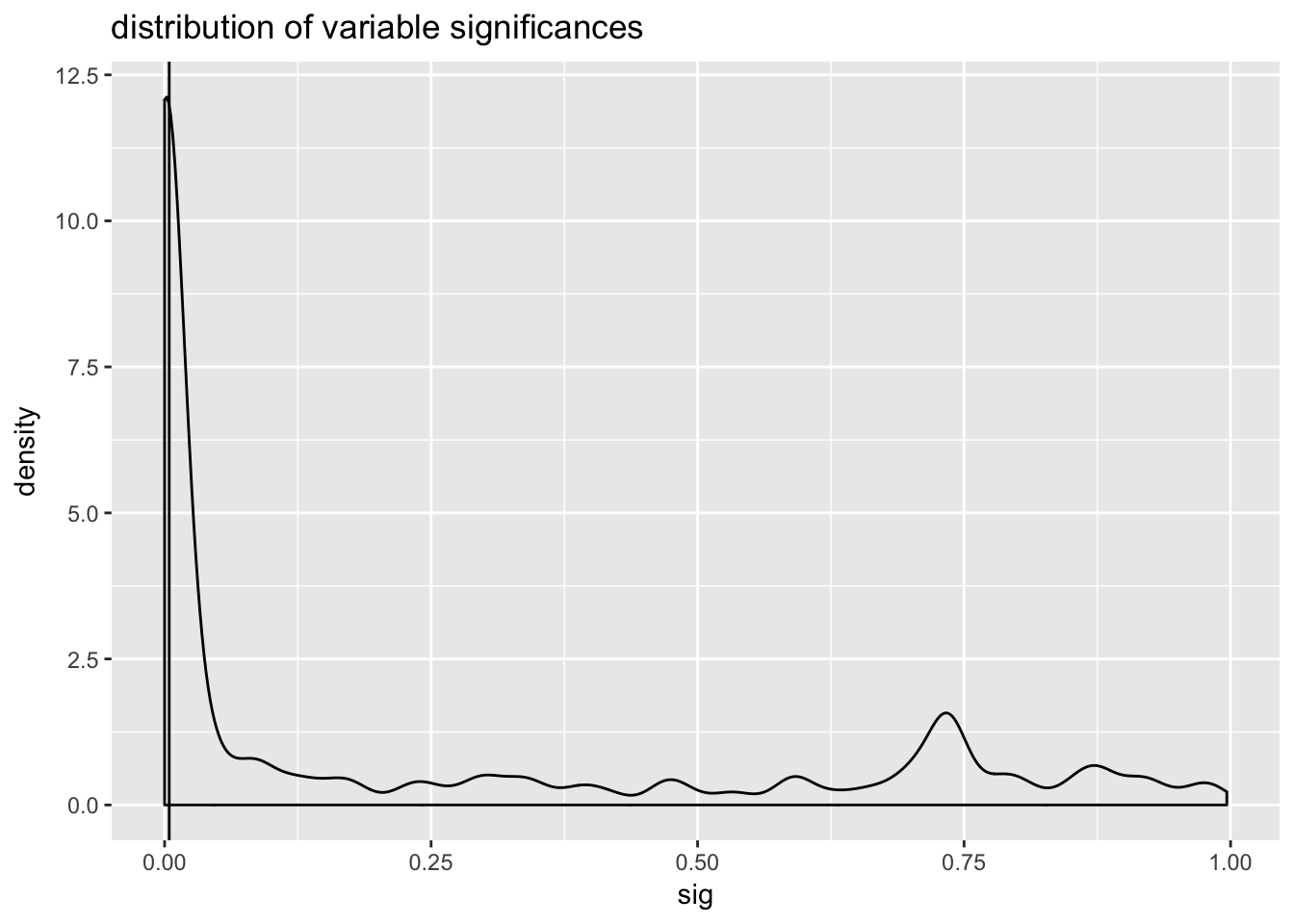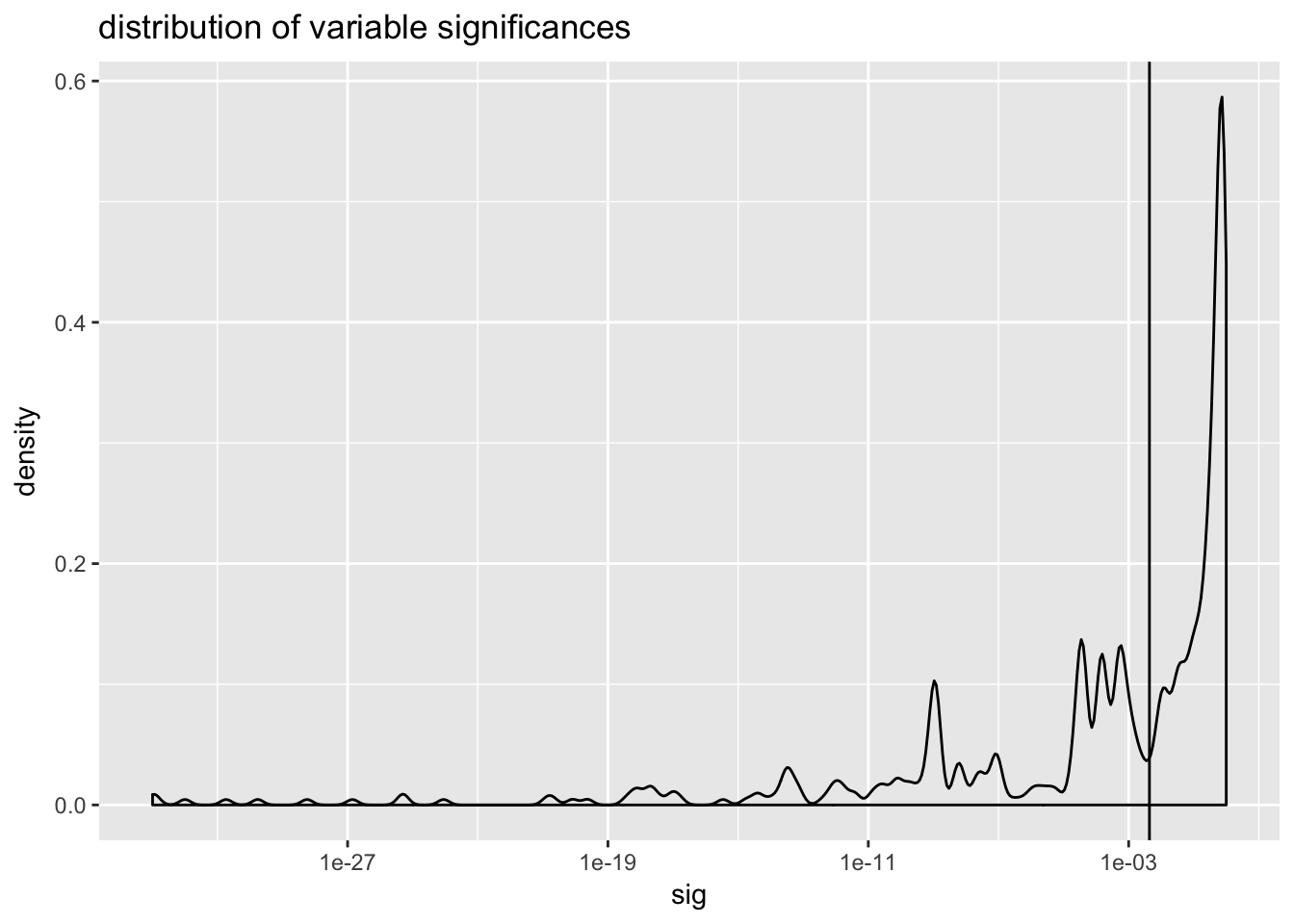``````#load some libraries
# https://github.com/WinVector/vtreat
library('vtreat')
packageVersion("vtreat")``````
``##  '1.0.3'``
``````# devtools::install_github("WinVector/WVPlots")
library('WVPlots')
library('parallel')
library('randomForest')``````
``## randomForest 4.6-12``
``## Type rfNews() to see new features/changes/bug fixes.``
``library('ggplot2')``
``````##
## Attaching package: 'ggplot2'``````
``````## The following object is masked from 'package:randomForest':
##
##     margin``````
``````# load the data as in the book
# change this path to match your directory structure
dir = '~/Documents/work/PracticalDataScienceWithR/zmPDSwR/KDD2009/'

stringsAsFactors=FALSE)
d\$churn = churn\$V1
d\$appetency = appetency\$V1
d\$upselling = upselling\$V1
set.seed(729375)
d\$rgroup = runif(dim(d)[])
dTrainM = subset(d,rgroup<=0.5)  # set for building models
dTrainC = subset(d,(rgroup>0.5) & (rgroup<=0.9)) # set for impact coding
dTest = subset(d,rgroup>0.9) # set for evaluation
rm(list=c('d','churn','appetency','upselling','dir'))
outcomes = c('churn','appetency','upselling')
vars = setdiff(colnames(dTrainM),
c(outcomes,'rgroup'))
yName = 'churn'
yTarget = 1``````
``````# build data treatments

set.seed(239525)

cl = parallel::makeCluster(4)

# build treatments on just the coding data
treatmentsC = designTreatmentsC(dTrainC,
vars,yName,yTarget,
smFactor=0.5,rareCount=2,rareSig=0.2,
parallelCluster=cl)``````
``````##  "vtreat 1.0.3 inspecting inputs Sat Feb 17 12:17:00 2018"
##  "designing treatments Sat Feb 17 12:17:00 2018"
##  " have initial level statistics Sat Feb 17 12:19:46 2018"
##  " scoring treatments Sat Feb 17 12:19:55 2018"
##  "have treatment plan Sat Feb 17 12:20:23 2018"
##  "rescoring complex variables Sat Feb 17 12:20:23 2018"
##  "done rescoring complex variables Sat Feb 17 12:23:35 2018"``````
``````kddSig = 1/length(vars)

ggplot(data=treatmentsC\$scoreFrame,aes(x=sig)) +
geom_vline(xintercept=kddSig) +
ggtitle("distribution of variable significances")````````````ggplot(data=treatmentsC\$scoreFrame,aes(x=sig)) +
scale_x_log10() +
ggtitle("distribution of variable significances")````````length(treatmentsC\$scoreFrame\$sig)``
``##  505``
``````selvars <- treatmentsC\$scoreFrame\$varName[treatmentsC\$scoreFrame\$sig<kddSig]
length(selvars)``````
``##  236``
``````trainP = prepare(treatmentsC,
dTrainM,
scale=TRUE,
varRestriction=selvars,
parallelCluster=cl)

testP = prepare(treatmentsC,
dTest,
scale=TRUE,
varRestriction=selvars,
parallelCluster=cl)

if(!is.null(cl)) {
parallel::stopCluster(cl)
cl = NULL
}``````
``````model <- randomForest(x=trainP[,selvars,drop=FALSE],
y=as.factor(as.character(trainP[[yName]])))
print(model)``````
``````##
## Call:
##  randomForest(x = trainP[, selvars, drop = FALSE], y = as.factor(as.character(trainP[[yName]])))
##                Type of random forest: classification
##                      Number of trees: 500
## No. of variables tried at each split: 15
##
##         OOB estimate of  error rate: 7.2%
## Confusion matrix:
##       -1 1  class.error
## -1 23202 5 0.0002154522
## 1   1796 6 0.9966703663``````
``````testP\$pred <- predict(model,newdata=testP[,selvars,drop=FALSE],
type='prob')[,as.character(yTarget),drop=TRUE]
testP[[yName]] = testP[[yName]]==yTarget
ti = 'RF prediction on test'
print(DoubleDensityPlot(testP, 'pred', yName,
title=ti))``````